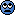# B Gram-Schimidt orthonormalization for three eigenvectors

#### Wrichik Basu

Gold Member
2018 Award
Say I have a matrix $A$ and it has three eigenvectors $|\psi_1\rangle$, $|\psi_2\rangle$ and $|\psi_3\rangle$. I want to orthogonalize these. Say my orthogonalized eigenvectors are $|\phi_1\rangle$, $|\phi_2\rangle$ and $|\phi_3\rangle$.
$$\begin{eqnarray} |\phi_1\rangle = \frac{|\psi_1\rangle}{|| \psi_1 ||} \\ \text{ } \nonumber \\ \phi_2 = \dfrac{ \left\{| \psi_2\rangle - \langle\phi_1 | \psi_2\rangle |\phi_1\rangle \right\} }{|| \{ \cdots \} ||} \\ \text{ } \nonumber \\ \phi_3 = \dfrac{ \left\{| \psi_3\rangle - \langle\phi_1 | \psi_3\rangle |\phi_1\rangle - \langle\phi_2 | \psi_3\rangle |\phi_2\rangle \right\} }{|| \{ \cdots \} ||} \end{eqnarray}$$
Our teacher didn't explain this at all. I learnt from this video, where the professor has done upto Eqn. (2) only. Can you check whether the third equation above is correct or not?

Last edited:
Related Classical Physics News on Phys.org

#### Michael Price

Say I have a matrix $A$ and it has three eigenvectors $|\psi_1\rangle$, $|\psi_1\rangle$ and $|\psi_1\rangle$. I want to orthogonalize these. Say my orthogonalized eigenvectors are $|\phi_1\rangle$, $|\phi_2\rangle$ and $|\phi_3\rangle$.
$$\begin{eqnarray} |\phi_1\rangle = \frac{|\psi_1\rangle}{|| \psi_1 ||} \\ \text{ } \nonumber \\ \phi_2 = \dfrac{ \left\{| \psi_2\rangle - \langle\phi_1 | \psi_2\rangle |\phi_1\rangle \right\} }{|| \{ \cdots \} ||} \\ \text{ } \nonumber \\ \phi_3 = \dfrac{ \left\{| \psi_3\rangle - \langle\phi_1 | \psi_3\rangle |\phi_1\rangle - \langle\phi_2 | \psi_3\rangle |\phi_2\rangle \right\} }{|| \{ \cdots \} ||} \end{eqnarray}$$
Our teacher didn't explain this at all. I learnt from this video, where the professor has done upto Eqn. (2) only. Can you check whether the third equation above is correct or not?
I suggest you edit your three original vectors.

#### Wrichik Basu

Gold Member
2018 Award
I suggest you edit your three original vectors.
You mean to say that I should write them such that they are orthogonal to each other?

#### kuruman

Homework Helper
Gold Member
You mean to say that I should write them such that they are orthogonal to each other?
Write them in such a way that they have different subscripts.#### Wrichik Basu

Gold Member
2018 Award
Write them in such a way that they have different subscripts.Went tangentially past my brain. How would different subscripts matter?

#### WWGD

Gold MemberWent tangentially past my brain. How would different subscripts matter?
You used the same subscript $_1$ for all three vectors.

•Wrichik Basu

#### kuruman

Homework Helper
Gold Member
Say I have a matrix $A$ and it has three eigenvectors $|\psi_1\rangle$, $|\psi_1\rangle$ and $|\psi_1\rangle$.
If they have the same subscript, they are the same vector - it's just a typo fix. You can check whether you have the correct expression for the third eigenvector by seeing if $\langle \phi_3|\phi_j\rangle\ = \delta_{3j}.$

#### Wrichik Basu

Gold Member
2018 Award
If they have the same subscript, they are the same vector - it's just a typo fix.
Fixed typo.

### Want to reply to this thread?

"Gram-Schimidt orthonormalization for three eigenvectors"

### Physics Forums Values

We Value Quality
• Topics based on mainstream science
• Proper English grammar and spelling
We Value Civility
• Positive and compassionate attitudes
• Patience while debating
We Value Productivity
• Disciplined to remain on-topic
• Recognition of own weaknesses
• Solo and co-op problem solving